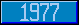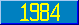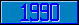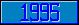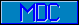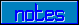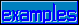## Scanning Algorithm (text)

### 1.1 Scanning Algorithm

A transition diagram is a network of no es and directed paths with at least one entrance node (indicated by the symbolor the symbol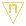, where n is an integer such as 1, 2, 3, etc.), , and at least one exit node (indicated by one of the following four symbols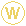,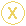,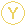,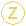;is the "normal" exit).

Each transition diagram defines a syntactic type, whose name is written in underscored lower-case letters, e.g., expr. A string being scanned through a "window" which looks at one character at a time is declared to be an instance of the syntactic type defined by a diagram if and only if the algorithm given below exactly scans the string while traversing the transition diagram from an entrance node to an exit node.

Each directed path from one node to another node is either associated with a symbol "on" that path, or the path is "blank". If a path has a symbol on it, the symbol can be either the name of a syntactic type defined by a transition diagram, implying a "call" (possibly recursive) to that diagram, or a symbol from the primitive alphabet. The primitive alphabet consists of the 95 ASCII graphics, including SP (space), plus ls, eol, eor, and eoi (the eoi character is used to indicate the end of argument or sub-argument level indirection; its actual form is not defined).

The scanning algorithm works as follows. In the next paragraph, the rule for following a path from one node to the next node is given. Once that can be done, the rule is applied iteratively starting at an entrance node until an exit node is encountered. At this point, a call to the diagram just traversed has been completed, and the path which made the call may be traversed. If a dead end is reached, and the window has not moved since entering the diagram, it only means that the call to this diagram has not succeeded and another path must be attempted. If a dead end is reached after moving the window over at least one input symbol since the entrance node, this is the indication of a syntax error.

The rule for leaving a node is as follows. All nonblank paths are tried, with primitive symbols tried first, then calls to other diagrams. The blank path, if it is present, provides the default case and is taken only after all other paths fail. A path with a primitive symbol on it may be traversed if and only if the symbol in the window equals the symbol on the path. In this case, the path is traversed and the window is moved one position to the right. A path with a call to a transition diagram may be traversed if and only if a call to that diagram results in successfully reaching an exit node of that diagram. Any window movement arising from a call to a diagram will be done within the called diagram.

Any path can direct the performance of an action, indicated by a number in a square box on the path. The action may be performed after the path is traversed. Certain actions, called "privileged actions", are always executed after traversing the path on which they appear; all other actions are executed only if both the semantic action flags Linact and Comact are True (see the scanning algorithm). Note that the actions within a called diagram precede the action specified on the path of the call.

If a diagram contains only one exit type, the X type is used. If it contains W, X, Y and/or Z, the exit actually used as a result of a given call may be tested by the caller. The notation used is as follows.

 single-exit diagram multiple-exit diagram

Actions generally make reference to temporary variables, such as A, B, C, D (see the diagram for the \$PIECE function). These variables are strictly local to each invocation of a diagram. A communication variable "Result" is used to pass values among diagrams.

Two branching notations are used in the diagrams. These are illustrated below.

Notation 1 indicates that this syntactic construct continues a theentrance of the named diagram. In other words, whenever the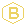(branch) symbol is encountered, the logical flow is transferred to the beginning of the diagram whose name appears to the right of the. This construct is merely a convenient notation for presentation of the diagrams. Notation 2 indicates a transfer of control to a specific entry point (other than theentrance) in the named diagram. The n in the symbolis an integer which indicates the number of the entry point in the diagram whose name appears to the right of the. This construct is used primarily to show clearly the control flow in the MUMPS language from DO, FOR, and XECUTE commands.

One other construct used in the diagrams is shown below.